Courses

# Test: Division - 2

## 10 Questions MCQ Test Mathematics (Maths) for Class 3 | Test: Division - 2

Description
This mock test of Test: Division - 2 for Class 3 helps you for every Class 3 entrance exam. This contains 10 Multiple Choice Questions for Class 3 Test: Division - 2 (mcq) to study with solutions a complete question bank. The solved questions answers in this Test: Division - 2 quiz give you a good mix of easy questions and tough questions. Class 3 students definitely take this Test: Division - 2 exercise for a better result in the exam. You can find other Test: Division - 2 extra questions, long questions & short questions for Class 3 on EduRev as well by searching above.
QUESTION: 1

Solution:
QUESTION: 2

Solution:

4 × 8 = 32
This implies,
32 ÷ 4 = 8

QUESTION: 3

### Ratna was reciting the 26 letters of the alphabet in groups of 3 : "ABC, DEF, GHI,....". Before she could finish, her friend Mohan said, "There will be 2 letters left over." Which of the following suggests that Mohan is correct?

Solution: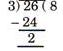∴ 26 divided by 3 leaves a remainder of 2.

QUESTION: 4

Amala has 47 pineapples to put in 5 baskets. She wants to put the same number of pineapples in each basket. If she evenly divides the pineapples among the 5 baskets, how many pineapples will be left over?

Solution: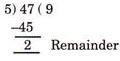QUESTION: 5

Estimate 756 ÷ 8. The quotient is between which two numbers?

Solution: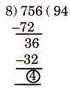∴  Quotient lies between 80 and 100.

QUESTION: 6

568 ÷ 4 = ________

Solution: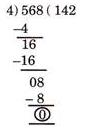QUESTION: 7

How many Rs. 5 coins will make a sum of Rs. 100?

Solution:

Rs. 100 ÷ Rs. 5 = 20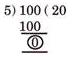QUESTION: 8

The remainder when 420 is divided by 8 is:

Solution: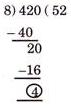∴ Remainder = 4

QUESTION: 9

Somya arranged some toys in the manner shown below: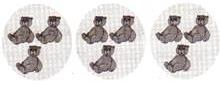Which of the following division facts is best represented by this grouping of toys?

Solution:
QUESTION: 10

A 36 cm long string is cut into 3 equal pieces. What is the length of each piece?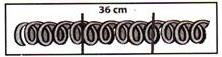Solution:

36 ÷ 3 = 12cm